GeeksforGeeks App
Open AppBrowser
Continue

# Motional Electromotive Force

The process of induction occurs when a change in magnetic flux causes an emf to oppose that change. One of the main reasons for the induction process in motion. We can say, for example, that a magnet moving toward a coil generates an emf, and that a coil moving toward a magnet creates a comparable emf.

This section will cover motion in a magnetic field that is stationary in relation to the planet Earth, resulting in motional emf. The Hall effect is one scenario where we may claim there is a motion that typically happens. The magnetic force experienced by moving charges in a magnetic field is indicated by

F = qvB sinθ

where F is the magnetic force, q is the charge, v is the velocity and B is the magnetic field.

### What is Lenz’s law?

Lenz’s law states that “The direction of the induced current is such that it opposes the change that has induced it.” i.e. when the magnetic flux through closed-loop changes, an electric current results in a direction so that it opposes the change that has induced it.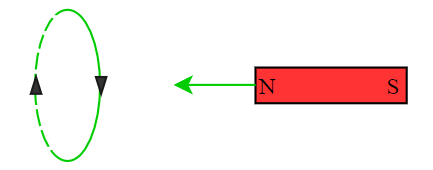As the magnet gets closer to the loop, the number of magnetic field lines passing through the area increases thus leading to an increase in the magnetic flux. So according to the law,  the induced current should weaken the flux. The field of the bar magnet is away from the magnet, so the induced field should be towards the magnet. Using the right-hand thumb rule, we find the direction of the current that produces a field towards a magnet i.e. north pole of the bar magnet faces the north pole of the loop. This is why we feel a slight force against the motion.

Example 1: In the given figure, the number of field lines passing through the loop increases. Find the direction of the induced current.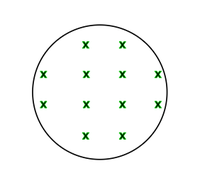We know that, the magnetic flux is:

φ = B . A

= B A cosθ,                                                                                                                                      (here θ = 0o)

where B is the magnetic field and A is the area of cross-section.

According to Lenz’s law, the direction of induced current should be in such a way that it opposes the change that induced it.

Here the change is increased in magnetic field . so in order to counter this, the induced magnetic field should be out of the plane. Using right-hand thumb rule, we get, The direction of induced current should be counter-clockwise/anti-clockwise direction.

Example 2: Consider the following figure where a conducting loop is placed near a long, straight wire carrying i. If the current increases continuously, find the direction of the induced current in the loop.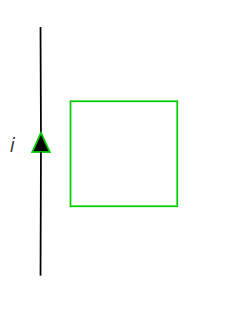Using right-hand thumb rule, we get the direction of the magnetic field produced due to the current-carrying wire being perpendicular to the loop and is going into the plane. Since the magnitude of the current is continuously increasing, the magnitude of the magnetic field also increases. Thus the flux through the loop increases.

So according to the Lenz’s law, the direction of induced current must be anti-clockwise to counter the change in the magnetic field.

### Faraday’s Law of Electromagnetic  Induction

Faraday after performing a number of experiments discovered the following law in nature: Whenever the flux of magnetic field through the area bounded by a closed conducting loop changes, an emf is produced in the loop.

The emf is given by,

ε = – dφ/dT

where φ =∫B . dS is the flux of the magnetic field through the area.

The SI unit of magnetic flux is called Weber which is equivalent to the Tesla meter. The -ve sign shows the direction of the induced current which we can find using Lenz’s law. The flux can be changed in a number of ways-

• By change in the magnitude of magnetic field B at the site of the loop.
• By change in the area of the loop
• By change in the angle between the area vector A and the field B.

Let us try to understand this through an experiment. A conducting rod AB of length l is moving on a rail with negligible resistance with velocity v in the uniform magnetic field B perpendicular to the plane of motion. We need to find out the current passing through the resistance R.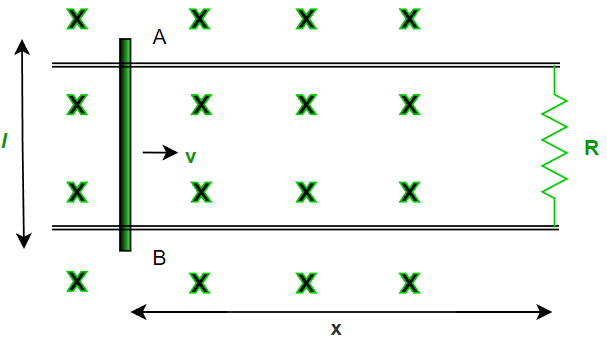Let x be the distance between the resistance and the rod at any timer t . According to faraday’s law, emf produced in a loop due to change in magnetic flux is,

ε = – dφ/dT

φt (flux at any time t) = B . A

= B l x

⇒ d (φt )/dt = d (Blx)/dt

= Bl dx/dt

= Blv                                                                                                                                              (since B and l are constants)

Therefore,

ε = – dφ/dT

= Blv

Using Ohm’s law: V = IR or I = V/R

Current through the resistance R is,

I = Blv/R                                                                                                                                                                (in a clockwise direction)

Amount of charge (q) passed through the loop in time ‘t’ = Δφ/R

where Δφ = Total change in flux in time ‘t’.

Example 1: The magnetic field into the plane of the loop is B(t) = 2t2. The cross-sectional area of the loop is 3 m2. Calculate the emf in the loop at time t = 3 seconds.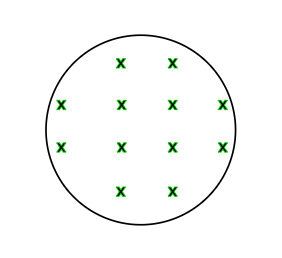Magnetic flux at any time t is , φ = B.A = 2t2.3 = 6t2 .

ε = – dφ/dT

= d(6t2)/dt

= 12t

EMF at t = 3sec ,

ε = 12(3)

= 36 volts.

Example 2: Considering the resistance of the loop to be 2 ohms, find the charge passed through the loop with reference to previous example.

Here, φ, at t = 0 = 2(0)=0.

And, φ, at t = 3 sec , = 2(3)2 = 18 .

Amount of charge (q) passed through the loop in time ‘t’ = Δφ/R = 18/2 = 9 Coulombs.

### Motional EMF

So what exactly is the external mechanism that maintains the electric field in the loop to drive the current? or, what is the mechanism to produce an emf? Let’s dive into this.

The magnetic flux φ =∫B . dS can be changed by –

1. Keeping the magnetic field constant as time passes and moving the whole part of the loop.
2. Keeping the loop at rest and changing the magnetic field.
3. Combination of both (1.) and (2.).

Fig.1 shows a rod PQ if length l moving in a magnetic field B with a constant velocity v. The length of the rod is perpendicular to the magnetic field and the velocity is perpendicular to both the magnetic field and the length of the rod.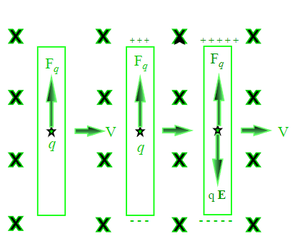At t=0 s, the free electrons of the wire also move with this velocity v together with the random velocity they have in the rod. As we know that the magnetic force due to the random velocity is zero on average. Thus the magnetic field exerts an average force of:

Fb = q(v x B)

where the charge on q is assumed as + 1.6 x 10-19. If we consider q as an electron, the magnitude of force remains the same but the direction reverses due to the negative charge in the electron.

This force is towards PQ and hence the electrons will move towards P. At time say t= ‘t’s, some amount of negative charge is accumulated at P and the positive charge appears at Q. So as a result of the accumulation of opposite charges at the endpoint, an electrostatic field E is developed within the wire from Q to P. The field exerts a force Fe = qE on each charge q.

The charge keeps on accumulating until a situation comes when Fb = Fe. Suppose at time t= T s, the equilibrium is achieved.

That means |q(v x B)| = |qE|

or

vB = E

After this, there is no resultant force on the free electrons of the wire PQ. The potential difference between the ends Q and P is,

V = El = vBl

Thus, it is the magnetic force on the moving free electrons that maintains the potential difference V = vBl and hence produces an emf ∈ = vBl.   As this emf is produced due to the motion of a conductor, it is called motional emf.

or more precisely,

∈ = (v x B) . l

i.e. the dot product of the length of rod l with the cross product of velocity v and magnetic field B. Using faraday’s law, we get the same result.

### Sample Problems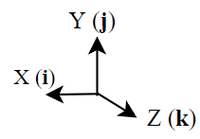for reference purposes

Problem 1: A rod PQ of length 10 m is moving with velocity 10 m/s in x axis under the influence of magnetic field of magnitude 8 T as shown in the fig. . Calculate the emf generated across its length after equilibrium is attained.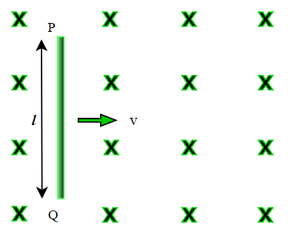We know that , v =10 i B = 8 and l = 10 j.   Since emf ∈ = (v x B) . l

So we will first find cross product of v and B.

(v x B) = (-10 i) x (8 k) =  80 j  , since i x k = -j

this implies that end P is of higher potential & Q is of lower potential as force on an electron will be downwards i.e. -j as              F =q(v x B).

EMF  ∈ = (v x B) . l = (80 j) . (10 j) = 800 V.     since j . j = 1

Therefore emf generated across the rod PQ is 800 V.

Problem 2: A rod PQ of length 1 m is moving with velocity 5 m/s at an angle of 37 from horizontal under the influence of magnetic field of magnitude 8 T as shown in the fig. . Calculate the emf generated across its length after equilibrium is attained.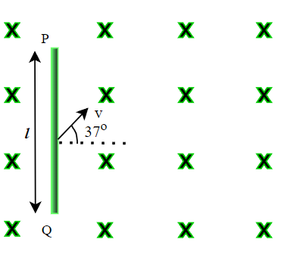We know that , v =(-5cos37oi + 5sin37o j) ,  B = 8 and l = 1 j.   Since emf ∈ = (v x B) . l

So we will first find cross product of v and B.

v x B = (-5cos37oi + 5sin37o j) × (8k) = (4 j + 3 i)              since cos 37 = 4/5 ,  sin 37 = 3/5 , i x k = -j and j x k = i

this implies that end P is of higher potential & Q is of lower potential  as         F =q(v x B).

EMF  ∈ = (v x B) . l = (4 j + 3 i) . (1 j) =  4 V.                       since  j . j = 1 and i . j = 0

Therefore emf generated across the rod PQ is 4 V.

Problem 3: A rod PQ of length 1 m is moving with velocity 10 m/s  horizontally under the influence of magnetic field of magnitude 8 T as shown in the fig. . Calculate the emf generated across its length after equilibrium is attained.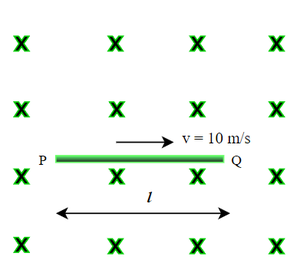We know that  v =-10 i ,  B = 8 k  and l =-1 i.       Since emf  ∈ = (v x B) .

v x B = -10 i x ( 8 k ) = 80 j

∈ = (v x B) . 80 j . (-1 i) = 0

As  we can see that the direction of v x B is perpendicular to the length of the rod l , emf generated will be zero.

That implies end P and Q are equipotential.

Problem 4: Consider a conducting rod of length 1 m (l = -1 j) , B = 1 i +2 j+3 k and v = 4 i +5 j+6 k . Calculate the emf generated within the rod.

We know that  v = 4 i +5 j+6 k ,  B = 1 i +2 j+3 k  and l =-1 j.   Since emf ∈ = (v x B) . l

So we will first find cross product of v and B.

(v x B) = (v = 4 i +5 j+6 k) x ( B = 1 i +2 j+3 k ) = 3 i – 6 j +3 k.

EMF  ∈ = (v x B) . l = ( 3 i – 6 j +3 k) . (-1 j) = 6V.

Therefore emf generated across the rod is 6 V.

Problem 5: A conducting rod AB of length l is hinged and rotated about end A, perpendicular to the uniform magnetic field B with constant angular velocity ω as shown. Calculate induced emf between two ends of the rod.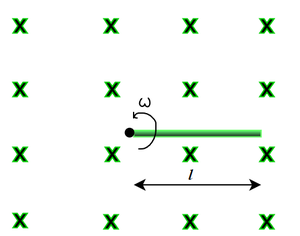As we move from A to B, the velocity of every other point on the rod is different. So we have to apply method of integration as we cannot apply the formula directly.

Let us consider an element of length dx at a distance from the end A

Therefore the velocity v of dx element is ωx as shown in fig.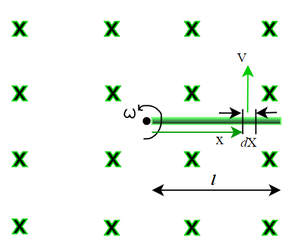so the emf induced in the element is dE = (v x B) . l  = B.ωx.dx . Therefore, we need to integrate dE through the length

∫dE = ∫B.ωx.dx

= B ω  0l  x . dx

E   = 1/2 B ω l2   V .

Therefore emf generated across the rod AB  is 1/2 B ω l2 V.

Problem 6: A conducting rod AB of length l = 1m  with end A fixed at the center and end B sliding over the circumference of a conducting loop of radius 1 m rotated with constant angular velocity ω = 4 radians/sec. A resistance r = 2 ohms is kept stationary with one end connected to A and other to the loop as shown in the figure. Calculate the current i that flows through the resistance. The magnitude of magnetic field is 2 T.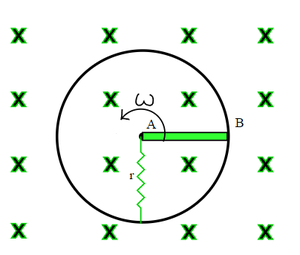As we have seen in problem no.5 , the emf induced across the ends of a rod moving with angular velocity ω with one of its end fixed is,-

ε = 1/2 B ω l2V.

i.e.  ε = 1/2 . 2. 4.1 = 4 V.     end A is of higher potential than B

Therefore potential difference across resistance r = 4 V

so using OHM’s law  , V = I.R, or I = V/R  = 4/2 = 2 A

i.e.  current through the resistance r is 2 amperes.

Problem 7: A rod of length l is moving with a velocity v making an angle of 37 degrees with its length. A uniform magnetic field B exists in a direction perpendicular to the plane of the motion. Calculate the induced emf in the rod.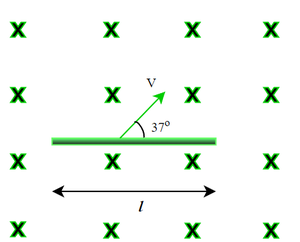We know that emf induced across the end of a rod moving with velocity v is – ∈ = (v x B) . l

i.e. the direction of v x B is 57 degrees clockwise from horizontal.

∈ = vBl cos (90 – 37) = vBl sin 37o  = 3 vBl/5   V,                 since the angle between v x B and l is 53 degrees.

Therefore emf induced across its ends is  3 vBl/5   Volts.

Problem 8: The given figure shows a wire AB of length l = 1m which can slide on a smooth rail of negligible resistance. The resistance of wire r = 1 ohm and external resistance R = 3 ohms. The wire is pulled to the right with constant speed v = 2 m/s. Calculate the amount of current flows through the wire. The magnitude of magnetic field is 2 T.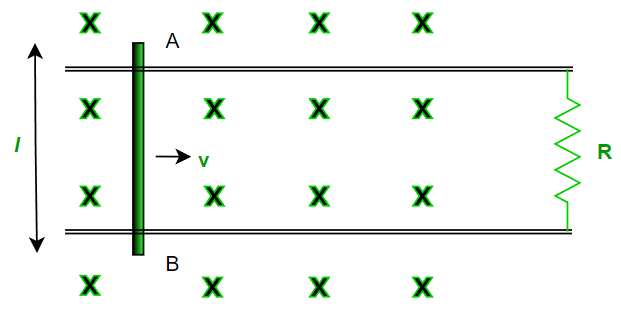METHOD -1

Emf induced across the end of a rod moving with velocity v is – ∈ = (v x B).l

i.e.   ∈ = 2. 2. 1 = 4 V.

Equivalent circuit diagram will be –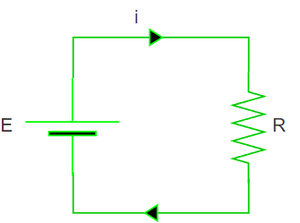Using ohm’s law , I = 4/(R+r) = 4/4 = 1 A.

Therefore current through the wire is 1 amperes.